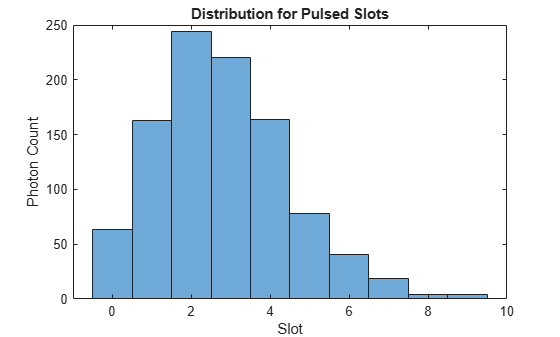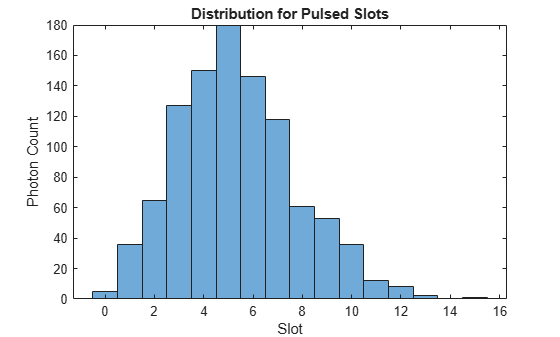# dsocPoissonChannel

Create a deep space optical communications Poisson channel

Since R2023a

## Description

The `dsocPoissonChannel` System object™ adds Poisson distributed noise to a signal through a deep space optical communications (DSOC) Poisson channel. The object uses the Poisson channel implementation described in section IV of the Interplanetary Network Progress report .

To add Poisson distributed noise to the input signal through a DSOC Poisson channel:

1. Create the `dsocPoissonChannel` object and set its properties.

2. Call the object with arguments, as if it were a function.

## Creation

### Syntax

``chan = dsocPoissonChannel``
``chan = dsocPoissonChannel(Name=Value)``

### Description

example

````chan = dsocPoissonChannel` creates a default DSOC Poisson channel System object.```

example

````chan = dsocPoissonChannel(Name=Value)` sets properties using one or more optional name-value arguments. For example, `dsocPoissonChannel(NumSignalPhotons=6)` sets the average number of signal photons per pulsed slot to `6`.```

## Properties

expand all

Unless otherwise indicated, properties are nontunable, which means you cannot change their values after calling the object. Objects lock when you call them, and the `release` function unlocks them.

If a property is tunable, you can change its value at any time.

Average signal photons per pulsed slot, specified as a nonnegative finite real scalar.

Data Types: `double`

Average background noise photons per slot, specified as nonnegative finite real scalar.

Data Types: `double`

Normalized timing offset, specified as a real number in the range [0, 1)

Data Types: `double`

Source of the random number stream, specified as `"Global stream"` or `"mt19937ar with seed"` .

• `"Global stream"`— The object uses the current global random number stream for uniformly and normally distributed random number generation.

• `"mt19937ar with seed"`— The object uses the mt19937ar algorithm for uniformly and normally distributed random number generation. In this case, the `reset` object function reinitializes the random number stream to the `Seed` value.

Data Types: `string` | `char`

Initial seed of the mt19937ar random number stream generator algorithm, specified as a nonnegative integer. When you call the `reset` object function, it reinitializes the mt19937ar random number stream to the `Seed` value.

Data Types: `double`

## Usage

### Syntax

``y = chan(x)``

### Description

````y = chan(x)` adds Poisson distributed noise to the input signal `x` through a DSOC Poisson channel, and returns the output signal `y`.```

### Input Arguments

expand all

Input signal, specified as an N-element column vector in which each value is a numeric `1` or `0`. N is the number of samples. The vector contains the modulated data, with `1` representing a laser pulse and `0` representing the absence of a laser pulse.

Data Types: `double`

### Output Arguments

expand all

Output signal, returned as an N-element column vector. N is the number of samples. The vector contains the estimate of the photon count within each slot. `y` is of the same length as `x`.

Data Types: `double`

## Object Functions

To use an object function, specify the System object as the first input argument. For example, to release system resources of a System object named `obj`, use this syntax:

`release(obj)`

expand all

 `step` Run System object algorithm `release` Release resources and allow changes to System object property values and input characteristics `clone` Create duplicate System object `isLocked` Determine if System object is in use `reset` Reset internal states of System object

## Examples

collapse all

To estimate photon count per slot at the receiver, configure a DSOC Poisson channel and pass the input signal through the channel.

Generate data.

```rng("default") symLen = 1000; m = 6; M = 2^m; % M-ary PPM data = randi([0 M-1],symLen,1);```

Create a default channel object.

`chanObj = dsocPoissonChannel;`

Modulate the data using the pulse position modulation (PPM) technique.

```modOut = zeros(symLen*M,1); mapIndex = (0:symLen-1)'*M + data + 1; % Mapping Index modOut(mapIndex) = 1;```

Pass the modulated data through a Poisson channel.

`receivedData = chanObj(modOut);`

Plot the distribution for pulsed slots.

```histogram(receivedData(modOut==1)) xlabel("Slot") ylabel("Photon Count") title("Distribution for Pulsed Slots")```Estimate photon counts per slot by configuring a DSOC Poisson channel and adding a timing offset to the signal.

Generate data.

```rng("default") symLen = 1000; m = 6; M = 2^m; % M-ary PPM data = randi([0 M-1],symLen,1);```

Create a DSOC Poisson channel object with these properties.

• Average signal photons per pulsed slot — `6`

• Average noise photons per slot — `0.01`

• Normalized timing offset — `0.11`

• Source of random number stream — `"mt19937ar with seed"`

• Initial seed of mt19937ar random number stream— ` 64`

```chanObj = dsocPoissonChannel(NumSignalPhotons=6,NumNoisePhotons=0.01,TimingOffset=0.11, ... RandomStream="mt19937ar with seed",Seed=64);```

Modulate the data using PPM.

```modOut = zeros(symLen*M,1); mapIndex = (0:symLen-1)'*M + data + 1; % Mapping Index modOut(mapIndex) = 1;```

Pass the modulated data through a Poisson channel.

`receivedData = chanObj(modOut);`

Plot the distribution for pulsed slots.

```histogram(receivedData(modOut==1)) xlabel("Slot") ylabel("Photon Count") title("Distribution for Pulsed Slots")```Moision, B., and J. Hamkins. "Coded Modulation for the Deep-Space Optical Channel: Serially Concatenated Pulse-Position Modulation." The Interplanetary Network Progress Report, vol. 42-161 (May 15, 2005): 1–25. https://ipnpr.jpl.nasa.gov/progress_report/42-161/161T.pdf.

 Quirk, Kevin J., Jonathan W. Gin, and Meera Srinivasan. "Optical PPM Synchronization for Photon Counting Receivers." In 2008 IEEE Military Communications Conference (MILCOM 2008), 1–7. San Diego, CA: IEEE, 2008. https://doi.org/10.1109/MILCOM.2008.4753054.